# Pipeline¶

Datashader provides a flexible series of processing stages that map from raw data into viewable images. As shown in the Introduction, using datashader can be as simple as calling datashade(), but understanding each of these stages will help you get the most out of the library.

The stages in a datashader pipeline are similar to those in a 3D graphics shading pipeline: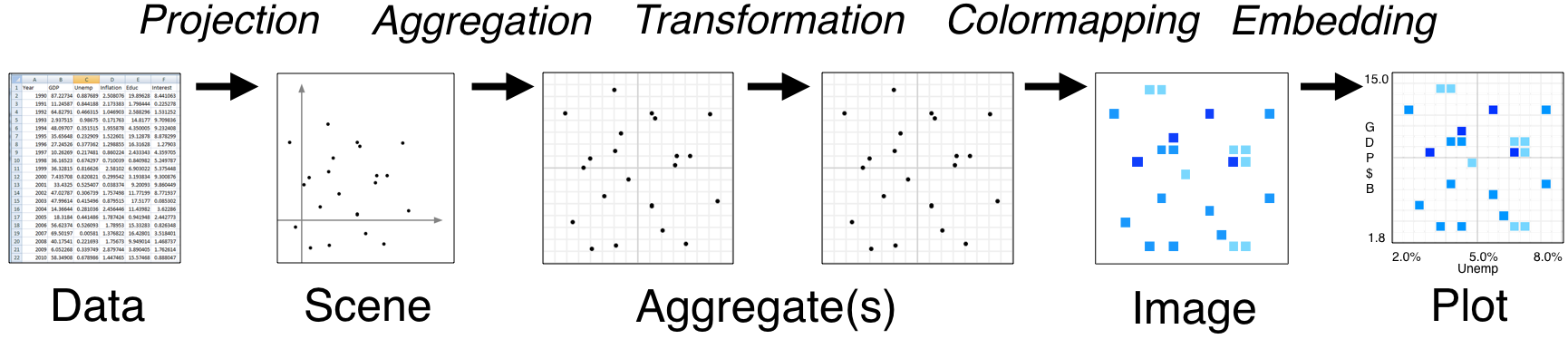Here the computational steps are listed across the top of the diagram, while the data structures or objects are listed along the bottom. Breaking up the computations in this way is what makes Datashader able to handle arbitrarily large datasets, because only one stage (Aggregation) requires access to the entire dataset. The remaining stages use a fixed-sized data structure regardless of the input dataset, allowing you to use any visualization or embedding methods you prefer without running into performance limitations.

In this notebook, we'll first put together a simple, artificial example to get some data, and then show how to configure and customize each of the data-processing stages involved:

## Data¶

For an example, we'll construct a dataset made of five overlapping 2D Gaussian distributions with different σs (spatial scales). By default we'll have 10,000 datapoints from each category, but you should see sub-second response times even for 1 million datapoints per category if you increase num.

In :
import pandas as pd
import numpy as np
from collections import OrderedDict as odict

num=10000
np.random.seed(1)

dists = {cat: pd.DataFrame(odict([('x',np.random.normal(x,s,num)),
('y',np.random.normal(y,s,num)),
('val',val),
('cat',cat)]))
for x,  y,  s,  val, cat in
[(  2,  2, 0.03, 10, "d1"),
(  2, -2, 0.10, 20, "d2"),
( -2, -2, 0.50, 30, "d3"),
( -2,  2, 1.00, 40, "d4"),
(  0,  0, 3.00, 50, "d5")] }

df = pd.concat(dists,ignore_index=True)
df["cat"]=df["cat"].astype("category")


Datashader can work many different data objects provided by different data libraries depending on the type of data involved, such as columnar data in Pandas or Dask dataframes, gridded multidimensional array data using xarray, columnar data on GPUs using cuDF, multidimensional arrays on GPUs using CuPy, and ragged arrays using SpatialPandas (see the Performance User Guide for a guide to selecting an appropriate library). Here, we're using a Pandas dataframe, with 50,000 rows by default:

In :
df.tail()

Out:
x y val cat
49995 -1.397579 0.610189 50 d5
49996 -2.649610 3.080821 50 d5
49997 1.933360 0.243676 50 d5
49998 4.306374 1.032139 50 d5
49999 -0.493567 -2.242669 50 d5

To illustrate this dataset, we'll make a quick-and-dirty Datashader plot that dumps these x,y coordinates into an image:

In :
import datashader as ds


CPU times: user 411 ms, sys: 11.9 ms, total: 423 ms
Wall time: 423 ms

Out: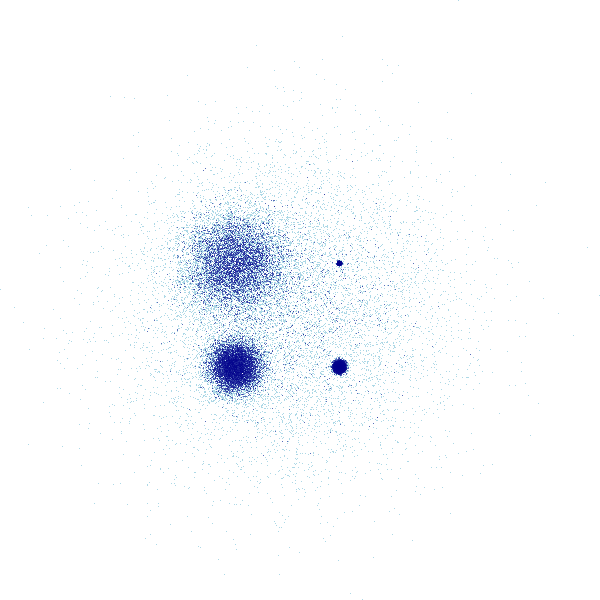Without any special tweaking, datashader is able to reveal the overall shape of this distribution faithfully: four summed 2D normal distributions of different variances, arranged at the corners of a square, overlapping another very high-variance 2D normal distribution centered in the square. This immediately obvious structure makes a great starting point for exploring the data, and you can then customize each of the various stages involved as described below.

Of course, this is just a static plot, and you can't see what the axes are, so we can instead embed this data into an interactive plot if we prefer:

Here, if you are running a live Python process, you can enable the "wheel zoom" tool on the right, zoom in anywhere in the distribution, and datashader will render a new image that shows the full distribution at that new location. If you are viewing this on a static web site, zooming will simply make the existing set of pixels larger, because this dynamic updating requires Python.

Now that you can see the overall result, we'll unpack each of the steps in the Datashader pipeline and show how this image is constructed from the data.

## Projection¶

Datashader is designed to render datasets projected on to a 2D rectangular grid, eventually generating an image where each pixel corresponds to one cell in that grid. The Projection stage is primarily conceptual, as it consists of you deciding what you want to plot and how you want to plot it:

• Variables: Select which variable you want to have on the x axis, and which one for the y axis. If those variables are not already columns in your dataframe (e.g. if you want to do a coordinate transformation), you'll need to create suitable columns mapping directly to x and y for use in the next step. For this example, the "x" and "y" columns are conveniently named x and y already, but any column name can be used for these axes.
• Ranges: Decide what ranges of those values you want to map onto the scene. If you omit the ranges, datashader will calculate the ranges from the data values, but you will often wish to supply explicit ranges for three reasons:
1. Calculating the ranges requires a complete pass over the data, which takes nearly as much time as actually aggregating the data, so your plots will be about twice as fast if you specify the ranges.
2. Real-world datasets often have some outliers with invalid values, which can make it difficult to see the real data, so after your first plot you will often want to specify only the range that appears to have valid data.
3. Over the valid range of data, you will often be mainly interested in a specific region, allowing you to zoom in to that area (though with an interactive plot you can always do that as needed).
• Axis types: Decide whether you want 'linear' or 'log' axes.
• Resolution: Decide what size of aggregate array you are going to want.

Here's an example of specifying a Canvas (a.k.a. "Scene") object for a 200x200-pixel image covering the range +/-8.0 on both axes:

In :
canvas = ds.Canvas(plot_width=300, plot_height=300,
x_range=(-8,8), y_range=(-8,8),
x_axis_type='linear', y_axis_type='linear')


At this stage, no computation has actually been done -- the canvas object is a purely declarative, recording your preferences to be applied in the next stage.

## Aggregation¶

Once a Canvas object has been specified, it can then be used to guide aggregating the data into a fixed-sized grid. You'll first need to know what your data points represent, i.e., what form each datapoint should take as it maps onto the rectangular grid. The library currently supports:

• Canvas.points: mapping each datapoint into the single closest grid cell to that datapoint's location
• Canvas.lines: mapping each datapoint into every grid cell falling between this point's location and the next.
• Canvas.raster: mapping each datapoint into an axis-aligned rectangle forming a regular grid with adjacent points.

Datashader can be extended to add additional types here and in each section below; see Extending Datashader for more details. Other plots like time series and network graphs are constructed out of these basic primitives.

### Reductions¶

One you have determined your mapping, you'll next need to choose a reduction operator to use when aggregating multiple datapoints into a given pixel. All of the currently supported reduction operators are incremental, which means that we can efficiently process datasets in a single pass. Given an aggregate bin to update (typically corresponding to one eventual pixel) and a new datapoint, the reduction operator updates the state of the bin in some way. (Actually, datapoints are normally processed in batches for efficiency, but it's simplest to think about the operator as being applied per data point, and the mathematical result should be the same.) A large number of useful reduction operators are supplied in ds.reductions, including:

count(column=None): increment an integer count each time a datapoint maps to this bin.

any(column=None): the bin is set to 1 if any datapoint maps to it, and 0 otherwise.

sum(column): add the value of the given column for this datapoint to a running total for this bin.

count_cat(column): given a bin with categorical data (i.e., Pandas' categorical datatype), count each category separately, adding the given datapoint to an appropriate category within this bin. These categories can later be collapsed into a single count if needed; see example below.

summary(name1=op1,name2=op2,...): allows multiple reduction operators to be computed in a single pass over the data; just provide a name for each resulting aggregate and the corresponding reduction operator to use when creating that aggregate.

The API documentation contains the complete list of reduction operators provided, including mean, min, max, var (variance), std (standard deviation). The reductions are also imported into the datashader namespace for convenience, so that they can be accessed like ds.mean() here.

For the operators above, those accepting a column argument will only do the operation if the value of that column for this datapoint is not NaN. E.g. count with a column specified will count the datapoints having non-NaN values for that column.

Once you have selected your reduction operator, you can compute the aggregation for each pixel-sized aggregate bin:

In :
canvas.points(df, 'x', 'y', agg=ds.count())

Out:
<xarray.DataArray (y: 300, x: 300)>
array([[0, 0, 0, ..., 0, 0, 0],
[0, 0, 0, ..., 0, 0, 0],
[0, 0, 0, ..., 0, 0, 0],
...,
[0, 0, 0, ..., 0, 0, 0],
[0, 0, 0, ..., 0, 0, 0],
[0, 0, 0, ..., 0, 0, 0]], dtype=int32)
Coordinates:
* x        (x) float64 -7.973 -7.92 -7.867 -7.813 ... 7.813 7.867 7.92 7.973
* y        (y) float64 -7.973 -7.92 -7.867 -7.813 ... 7.813 7.867 7.92 7.973

The result of will be an xarray DataArray data structure containing the bin values (typically one value per bin, but more for multiple category or multiple-aggregate operators) along with axis range and type information.

We can visualize this array in many different ways by customizing the pipeline stages described in the following sections, but for now we'll use HoloViews to render images using the default parameters to show the effects of a few different aggregate operators:

In :
tf.Images(tf.shade(   canvas.points(df,'x','y', ds.count()),     name="count()"),

Out:
 count()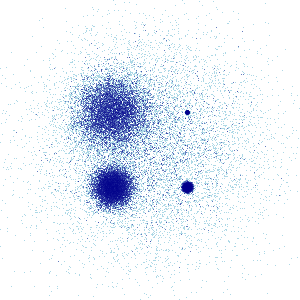any()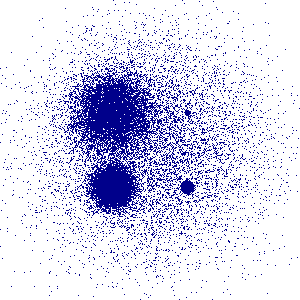mean('y')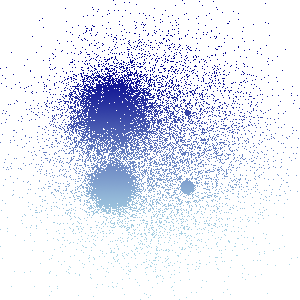50- mean('val')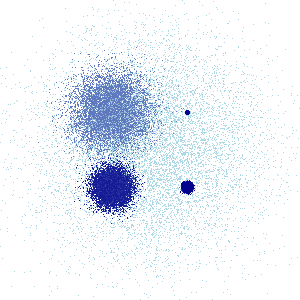Here count() renders each bin's count in a different color, to show the true distribution, while any() turns on a pixel if any point lands in that bin, and mean('y') averages the y column for every datapoint that falls in that bin. Of course, since ever datapoint falling into a bin happens to have the same y value, the mean reduction with y simply scales each pixel by its y location.

For the last image above, we specified that the val column should be used for the mean reduction, which in this case results in each category being assigned a different color, because in our dataset all items in the same category happen to have the same val. Here we also manipulated the result of the aggregation before displaying it by subtracting it from 50, as detailed in the next section.

## Transformation¶

Now that the data has been projected and aggregated into a gridded data structure, it can be processed in any way you like, before converting it to an image as will be described in the following section. At this stage, the data is still stored as bin data, not pixels, which makes a very wide variety of operations and transformations simple to express.

For instance, instead of plotting all the data, we can easily plot only those bins in the 99th percentile by count (left), or apply any NumPy ufunc to the bin values (whether or not it makes any sense!:

In :
agg  = canvas.points(df, 'x', 'y')


Out:
 99th Percentile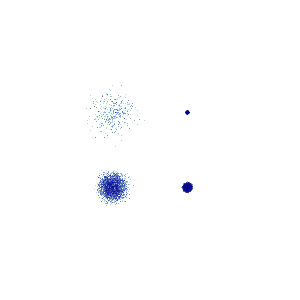Numpy square ufuncNumpy sin ufunc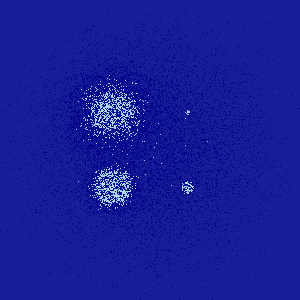The xarray documentation describes all the various transformations you can apply from within xarray, and of course you can always extract the data values and operate on them outside of xarray for any transformation not directly supported by xarray, then construct a suitable xarray object for use in the following stage. Once the data is in the aggregate array, you generally don't have to worry much about optimization, because it's a fixed-sized grid regardless of your data size, and so it is very straightforward to apply arbitrary transformations to the aggregates.

The above examples focus on a single aggregate, but there are many ways that you can use multiple data values per bin as well. For instance, if you collect categorical data, you will have an aggregate value for each category for each bin:

In :
aggc = canvas.points(df, 'x', 'y', ds.count_cat('cat'))
aggc

Out:
<xarray.DataArray (y: 300, x: 300, cat: 5)>
array([[[0, 0, 0, 0, 0],
[0, 0, 0, 0, 0],
[0, 0, 0, 0, 0],
...,
[0, 0, 0, 0, 0],
[0, 0, 0, 0, 0],
[0, 0, 0, 0, 0]],

[[0, 0, 0, 0, 0],
[0, 0, 0, 0, 0],
[0, 0, 0, 0, 0],
...,
[0, 0, 0, 0, 0],
[0, 0, 0, 0, 0],
[0, 0, 0, 0, 0]],

[[0, 0, 0, 0, 0],
[0, 0, 0, 0, 0],
[0, 0, 0, 0, 0],
...,
[0, 0, 0, 0, 0],
[0, 0, 0, 0, 0],
[0, 0, 0, 0, 0]],

...,

[[0, 0, 0, 0, 0],
[0, 0, 0, 0, 0],
[0, 0, 0, 0, 0],
...,
[0, 0, 0, 0, 0],
[0, 0, 0, 0, 0],
[0, 0, 0, 0, 0]],

[[0, 0, 0, 0, 0],
[0, 0, 0, 0, 0],
[0, 0, 0, 0, 0],
...,
[0, 0, 0, 0, 0],
[0, 0, 0, 0, 0],
[0, 0, 0, 0, 0]],

[[0, 0, 0, 0, 0],
[0, 0, 0, 0, 0],
[0, 0, 0, 0, 0],
...,
[0, 0, 0, 0, 0],
[0, 0, 0, 0, 0],
[0, 0, 0, 0, 0]]], dtype=int32)
Coordinates:
* x        (x) float64 -7.973 -7.92 -7.867 -7.813 ... 7.813 7.867 7.92 7.973
* y        (y) float64 -7.973 -7.92 -7.867 -7.813 ... 7.813 7.867 7.92 7.973
* cat      (cat) <U2 'd1' 'd2' 'd3' 'd4' 'd5'

Currently only counts are supported for categories, but other reduction operators can be implemented as well (a to-do item).

You can then select a specific category or subset of them for further processing, where .sum(dim='cat') will collapse across such a subset to give a single aggregate array:

In :
agg_d3_d5=aggc.sel(cat=['d3', 'd5']).sum(dim='cat')


Out:
 Category d3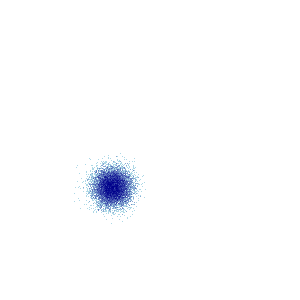Categories d3 and d5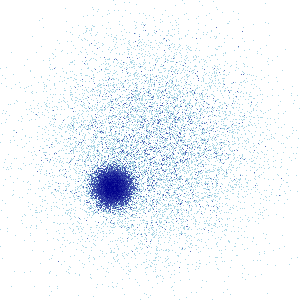You can also combine multiple aggregates however you like, as long as they were all constructed using the same Canvas object (which ensures that their aggregate arrays are the same size) and cover the same axis ranges:

In :
tf.Images(tf.shade(agg_d3_d5.where(aggc.sel(cat='d3') == aggc.sel(cat='d5')), name="d3+d5 where d3==d5"),
tf.shade(      agg.where(aggc.sel(cat='d3') == aggc.sel(cat='d5')), name="d1+d2+d3+d4+d5 where d3==d5"))

Out:
 d3+d5 where d3==d5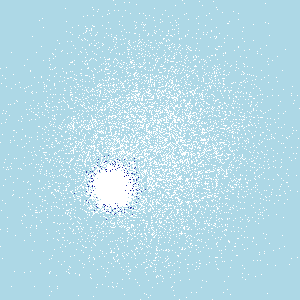d1+d2+d3+d4+d5 where d3==d5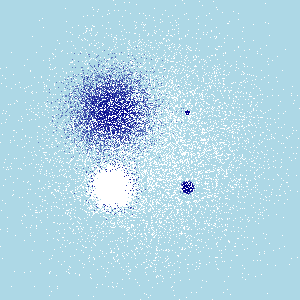The above two results are using the same mask (only those bins where the counts for 'd3' and 'd5' are equal), but applied to different aggregates (either just the d3 and d5 categories, or the entire set of counts).

## Colormapping¶

As you can see above, the usual way to visualize an aggregate array is to map from each array bin into a color for a corresponding pixel in an image. The above examples use the tf.shade() method, which maps a scalar aggregate bin value into an RGB (color) triple and an alpha (opacity) value. By default, the colors are chosen from the colormap ['lightblue','darkblue'] (i.e., #ADD8E6 to #00008B), with intermediate colors chosen as a linear interpolation independently for the red, green, and blue color channels (e.g. AD to 00 for the red channel, in this case). The alpha (opacity) value is set to 0 for empty bins and 1 for non-empty bins, allowing the page background to show through wherever there is no data. You can supply any colormap you like, including Bokeh palettes, Matplotlib colormaps, or a list of colors (using the color names from ds.colors, integer triples, or hexadecimal strings):

In :
from bokeh.palettes import RdBu9
tf.shade(agg,cmap=[(230,230,0), "orangered", "#300030"], name="yellow, orange red, dark purple"),

Out:
 darkred, yellow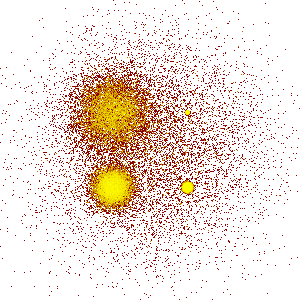yellow, orange red, dark purple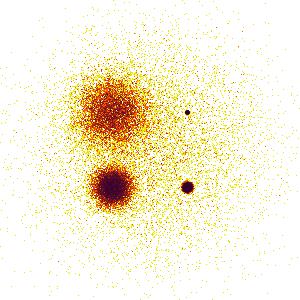Bokeh RdBu9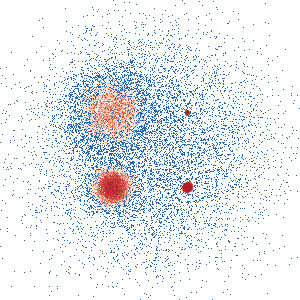#### Colormapping categorical data¶

If you want to use tf.shade with a categorical aggregate, you can use a colormap just as for a non-categorical aggregate if you first select a single category using something like aggc.sel(cat='d3') or else collapse all categories into a single aggregate using something like aggc.sum(dim='cat'). Or you can instead use tf.shade with the categorical aggregate directly, which will assign a color to each category and then mix the colors according to the values of each category:

In :
color_key = dict(d1='blue', d2='green', d3='red', d4='orange', d5='purple')


Out:
 Default color key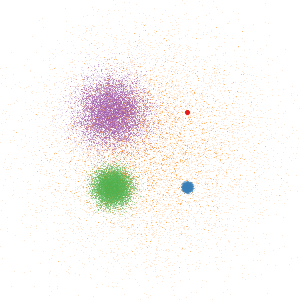Custom color key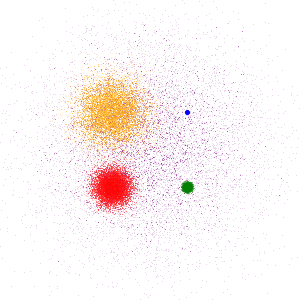Here the different colors mix not just on the page, but per pixel, with pixels having non-zero counts from multiple categories taking intermediate color values. The actual data values are used to calculate the alpha channel, with this computed color being revealed to a greater or lesser extent depending on the value of the aggregate for that bin. The default color key for categorical data provides distinguishable colors for a couple of dozen categories, but you can provide an explicit color_key if you prefer.

Choosing colors for different categories is more of an art than a science, because the colors not only need to be distinguishable, their combinations also need to be distinguishable if those categories ever overlap in nearby pixels, or else the results will be ambiguous. In practice, only a few categories can be reliably distinguished in this way, but zooming in (as shown below) can be used to help disambiguate overlapping colors, as long as the basic set of colors is itself distinguishable.

#### Transforming data values for colormapping¶

In each of the above examples, you may have noticed that we never needed to specify any parameters about the data values; the plots just appear like magic. That magic is implemented in tf.shade. What tf.shade does for a 2D aggregate (non-categorical) is:

1. Mask out all bins with a NaN value (for floating-point arrays) or a zero value (for integer arrays); these bins will not have any effect on subsequent computations. Unfortunately, integer arrays do not support NaN; using zero as a pseudo-NaN works well for counts but not for all integer data, which is something that may need to be generalized in a future version of the library (a to-do item).

2. Transform the bin values using a specified scalar function how. Calculates the value of that function for the difference between each bin value and the minimum non-masked bin value. E.g. for how="linear", simply returns the difference unchanged. Other how functions are discussed below.

3. Map the resulting transformed data array into the provided colormap. First finds the value span (l,h) for the resulting transformed data array -- what are the lowest and highest non-masked values? -- and then maps the range (l,h) into the full range of the colormap provided. Masked values are given a fully transparent alpha value, and non-masked ones are given a fully opaque alpha value.

The result is thus auto-ranged to show whatever data values are found in the aggregate bins (though the span argument can be used to set the range explicitly where appropriate).

As described in plotting_pitfalls.ipynb, auto-ranging is only part of what is required to reveal the structure of the dataset; it's also crucial to automatically and potentially nonlinearly map from the aggregate values (e.g. bin counts) into the colormap. If we used a linear mapping, we'd see very little of the structure of the data:

In :
tf.shade(agg,how='linear')

Out: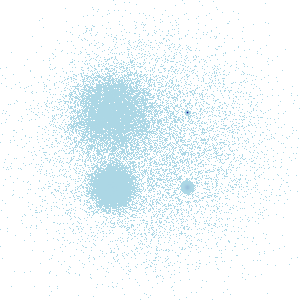In the linear version, you can see that the bins that have zero count show the background color, since they have been masked out using the alpha channel of the image, and that the rest of the pixels have been mapped to colors near the bottom of the colormap. If you peer closely at it, you may even be able to see that one pixel (from the smallest Gaussian) has been mapped to the highest color in the colormap (here dark blue). But no other structure is visible, because the highest-count bin is so much higher than all of the other bins:

In :
top15=agg.values.flat[np.argpartition(agg.values.flat, -15)[-15:]]
print(sorted(top15))
print(sorted(np.round(top15*255.0/agg.values.max()).astype(int)))

[342, 345, 345, 351, 355, 357, 363, 392, 399, 405, 1075, 1148, 1169, 1172, 3918]
[22, 22, 22, 23, 23, 23, 24, 26, 26, 26, 70, 75, 76, 76, 255]


I.e., if using a colormap with 255 colors, the largest bin (agg.values.max()) is mapped to the highest color, but with a linear scale all of the other bins map to only the first 24 colors, leaving all intermediate colors unused. If we want to see any structure for these intermediate ranges, we need to transform these numerical values somehow before displaying them. For instance, if we take the logarithm of these large values, they will be mapped into a more tractable range:

In :
print(np.log1p(sorted(top15)))

[5.83773045 5.84643878 5.84643878 5.86363118 5.87493073 5.88053299
5.89715387 5.97380961 5.99146455 6.00635316 6.98100574 7.04664728
7.06475903 7.06731985 8.2735918 ]


So we can plot the logarithms of the values (how='log', below), which is an arbitrary transform but is appropriate for many types of data. Alternatively, we can make a histogram of the numeric values, then assign a pixel color to each equal-sized histogram bin to ensure even usage of every displayable color (how='eq_hist'; see plotting pitfalls. We can even supply any arbitrary transformation to the colormapper as a callable, such as a twenty-third root:

In :
tf.Images(tf.shade(agg,how='log', name="log"),
tf.shade(agg,how=lambda d, m: np.where(m, np.nan, d)**(1/23.), name="23rd root"))

Out:
 log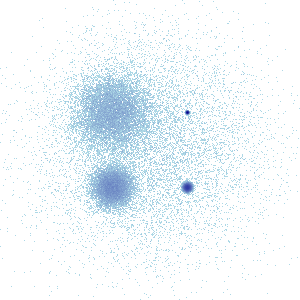eq_hist23rd root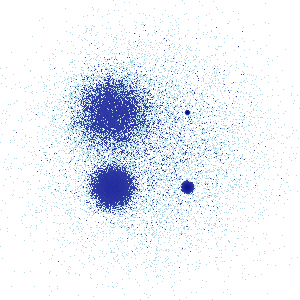Usually, however, such custom operations are done directly on the aggregate during the Transformation stage; the how operations are meant for simple, well-defined transformations solely for the final steps of visualization, which allows the main aggregate array to stay in the original units and scale in which it was measured. Using how also helps simplify the subsequent Embedding stage, letting it provide one of a fixed set of legend types, either linear (for how=linear), logarithmic (for how=log) or percentile (for how=eq_hist). See the shade docs for more details on the how functions. The shade function applies the how method similarly for categorical aggregates, based on the total across all categories, but then uses it for the alpha (opacity) channel of the image, rather than to index into a separate colormap.

Once an image has been created, it can be further transformed with a set of functions from ds.transfer_functions.

For instance, because it can be difficult to see individual dots, particularly for zoomed-in plots, you can transform the image to replace each non-transparent pixel with a shape, such as a circle (default) or square. This process is called spreading:

In :
img = tf.shade(aggc, name="Original image")

tf.Images(img,

Out:
 Original imagespread 1px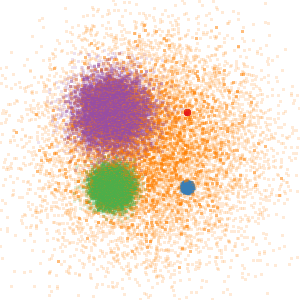spread 2px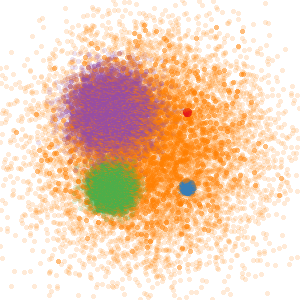spread square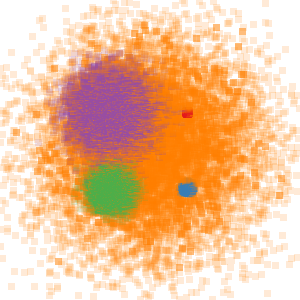As you can see, spreading is very effective for isolated datapoints, which is what it's normally used for, but it has overplotting-like effects for closely spaced points like in the green and purple regions above, and so it would not normally be used when the datapoints are dense.

Spreading can be used with a custom mask, as long as it is square and an odd width and height (so that it will be centered over the original pixel):

In :
mask = np.array([[1, 1, 1, 1, 1],
[1, 0, 0, 0, 1],
[1, 0, 0, 0, 1],
[1, 0, 0, 0, 1],
[1, 1, 1, 1, 1]])